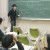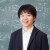Research Fields
Mathematics, Index Theorem
Term and History
2017/04/01 - 2020/03/31 Research Scientist
2020/06/01 - 2023/03/31 Visiting Scientist (Main: Lecturer, Department of Mathematical Sciences, Shinshu University)
2023/05/01 - Visiting Scientist (Main: Associate Professor, Graduate School of Science, Kyoto University)

## Self-introduction

I am Yosuke KUBOTA, a mathematician who have joined the iTHEMS from 2017. I'm basically working in the area of mathematics called index theory or noncommutative geometry (NCG), which has developed highly abstract theory related with a wide variety of other mathematics such as operator algebra, topology, geometry, group theory and mathematical physics. For example, a mathematics of topological insulators is one of the branches of NCG. I aspire to apply the theory of NCG to practical problems in mathematics and other areas. Please feel free to talk to me anytime.

## Related Events### Theory of Operator Algebras (9th)

March 5 (Tue) at 15:30 - 16:30, 2019 Math Lecture Theory of Operator Algebras### Theory of Operator Algebras (8th)

March 5 (Tue) at 14:00 - 15:00, 2019 Math Lecture Theory of Operator Algebras### Theory of Operator Algebras (7th)

January 10 (Thu) at 10:30 - 12:00, 2019 Math Lecture Theory of Operator Algebras### Theory of Operator Algebras (6th)

December 20 (Thu) at 15:30 - 17:00, 2018 Math Lecture Theory of Operator Algebras### Theory of Operator Algebras (5th)

November 8 (Thu) at 13:30 - 15:00, 2018 Math Lecture Theory of Operator Algebras### Theory of Operator Algebras (4th)

October 18 (Thu) at 15:30 - 17:00, 2018 Math Lecture Theory of Operator Algebras### Theory of Operator Algebras (3rd)

July 12 (Thu) at 15:30 - 17:00, 2018 Math Lecture Theory of Operator Algebras### Theory of Operator Algebras (2nd)

June 21 (Thu) at 15:30 - 17:00, 2018 Math Lecture Theory of Operator Algebras### Theory of Operator Algebras (1st)

May 10 (Thu) at 15:30 - 16:30, 2018 Math Lecture Theory of Operator Algebras### Topology of linear operators and topological insulators

December 27 (Wed) at 10:30 - 11:30, 2017 Seminar SUURI-COOL Seminar SUURI-COOL (Kyoto)### The Riemann-Roch theorem

September 26 (Tue) at 14:00 - 15:00, 2017 Seminar